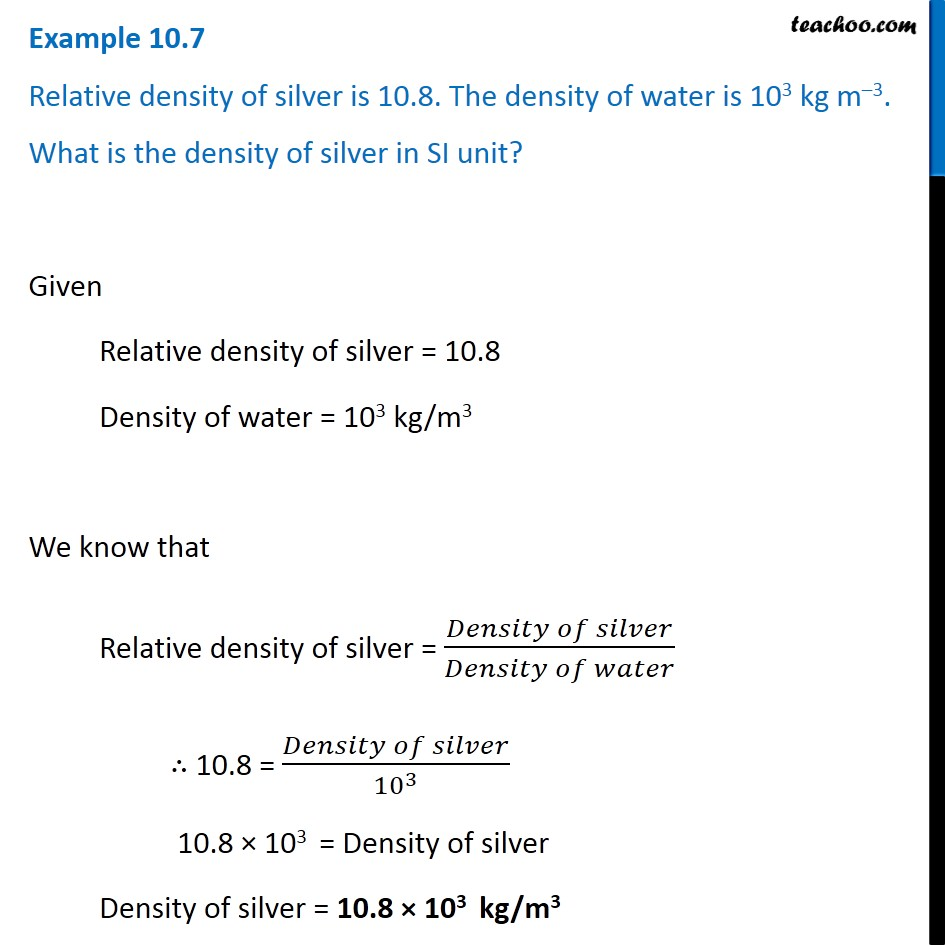Examples from NCERT Book

Class 9
Chapter 10 Class 9 - GravitationLearn in your speed, with individual attention - Teachoo Maths 1-on-1 Class

### Transcript

Example 10.7 Relative density of silver is 10.8. The density of water is 103 kg m–3. What is the density of silver in SI unit? Given Relative density of silver = 10.8 Density of water = 103 kg/m3 We know that Relative density of silver = (𝐷𝑒𝑛𝑠𝑖𝑡𝑦 𝑜𝑓 𝑠𝑖𝑙𝑣𝑒𝑟)/(𝐷𝑒𝑛𝑠𝑖𝑡𝑦 𝑜𝑓 𝑤𝑎𝑡𝑒𝑟) ∴ 10.8 = (𝐷𝑒𝑛𝑠𝑖𝑡𝑦 𝑜𝑓 𝑠𝑖𝑙𝑣𝑒𝑟)/〖10〗^3 10.8 × 103 = Density of silver Density of silver = 10.8 × 103 kg/m3